48

14

23

27

# Color

This library provides a wide array of functions for dealing with color. This includes conversion between colorspaces, measuring distance between colors, simulating color blindness, and generating color scales for graphics, among other things.

# This package is deprecated---use Colors.jl instead

This package was the original Julia package for handling colors, but several backwardly-incompatible changes were necessary, and a new package Colors.jl was forked from this one. This package will not be installable on future versions of julia, so all users should migrate to Colors.jl.

## Colorspaces

What follows is a synopsis of every colorspace implemented in Color.jl. Any color value can be converted to a similar value in any other colorspace using the `convert` function.

E.g.

``````convert(RGB, HSL(270, 0.5, 0.5))
``````

Depending on the source and destination colorspace, this may not be perfectly lossless.

### RGB

The sRGB colorspace.

``````immutable RGB{T} <: ColorValue
r::T # Red in [0,1]
g::T # Green in [0,1]
b::T # Blue in [0,1]
end
``````

RGBs may be defined with two broad number types: `FloatingPoint` and `FixedPoint`. `FixedPoint` come from the `FixedPointNumbers` package, and represent fractional numbers (between 0 and 1, inclusive) internally using integers. For example, `0xffuf8` creates a `Ufixed8` number with value equal to `1.0` but which internally is represented as `0xff`. This strategy ensures that `1` always means "saturated color", regardless of how that value is represented. Ordinary integers should not be used, although the convenience constructor `RGB(1,0,0)` will create a value `RGB{Float64}(1.0, 0.0, 0.0)`.

The parametric representation of colors facilitates interfacing with external libraries that may require a specific representation. It's also worth nothing that this package defines an `AbstractRGB{T}` type, from which you can define your own variants of RGB. For example, if you need a `BGR{Ufixed8}<:AbstractRGB{Ufixed8}` type to interface with a C library, you can define this easily. See an example of this in the `test/layout.jl` file.

If you do define your own `AbstractRGB`, note that the constructor must initialize the values in the order `(r,g,b)` regardless of how they are arranged internally in memory.

### HSV

Hue-Saturation-Value. A common projection of RGB to cylindrical coordinates. This is also sometimes called "HSB" for Hue-Saturation-Brightness.

``````immutable HSV{T} <: ColorValue
h::T # Hue in [0,360]
s::T # Saturation in [0,1]
v::T # Value in [0,1]
end
``````

`T` must be of `FloatingPoint` type, since the values range beyond what can be represented with most `FixedPoint` types.

### HSL

Hue-Saturation-Lightness. Another common projection of RGB to cylindrical coordinates.

``````immutable HSL{T} <: ColorValue
h::T # Hue in [0,360]
s::T # Saturation in [0,1]
l::T # Lightness in [0,1]
end
``````

### XYZ

The XYZ colorspace standardized by the CIE in 1931, based on experimental measurements of color perception culminating in the CIE standard observer (see `cie_color_match`)

``````immutable XYZ{T} <: ColorValue
x::T
y::T
z::T
end
``````

Currently, XYZ is the only type other than RGB supporting `FixedPoint`.

### xyY

The xyY colorspace is another CIE standardized color space, based directly off of a transformation from XYZ. It was developed specifically because the xy chromaticity space is invariant to the lightness of the patch.

``````immutable xyY{T} <: ColorValue
x::T
y::T
Y::T
end
``````

### LAB

A perceptually uniform colorpsace standardized by the CIE in 1976. See also LUV, the associated colorspace standardized the same year.

``````immutable LAB{T} <: ColorValue
l::T # Luminance in approximately [0,100]
a::T # Red/Green
b::T # Blue/Yellow
end
``````

### LUV

A perceptually uniform colorpsace standardized by the CIE in 1976. See also LAB, a similar colorspace standardized the same year.

``````immutable LUV{T} <: ColorValue
l::T # Luminance
u::T # Red/Green
v::T # Blue/Yellow
end
``````

### LCHab

The LAB colorspace reparameterized using cylindrical coordinates.

``````immutable LCHab{T} <: ColorValue
l::T # Luminance in [0,100]
c::T # Chroma
h::T # Hue in [0,360]
end
``````

### LCHuv

The LUV colorspace reparameterized using cylindrical coordinates.

``````immutable LCHuv{T} <: ColorValue
l::T # Luminance
c::T # Chroma
h::T # Hue
end
``````

### DIN99

The DIN99 uniform colorspace as described in the DIN 6176 specification.

``````immutable DIN99{T} <: ColorValue
l::T # L99 (Lightness)
a::T # a99 (Red/Green)
b::T # b99 (Blue/Yellow)
end
``````

### DIN99d

The DIN99d uniform colorspace is an improvement on the DIN99 color space that adds a correction to the X tristimulus value in order to emulate the rotation term present in the DeltaE2000 equation.

``````immutable DIN99d{T} <: ColorValue
l::T # L99d (Lightness)
a::T # a99d (Reddish/Greenish)
b::T # b99d (Bluish/Yellowish)
end
``````

### DIN99o

Revised version of the DIN99 uniform colorspace with modified coefficients for an improved metric. Similar to DIN99d X correction and the DeltaE2000 rotation term, DIN99o achieves comparable results by optimized `a*/b*` rotation and chroma compression terms.

``````immutable DIN99o{T} <: ColorValue
l::T # L99o (Lightness)
a::T # a99o (Red/Green)
b::T # b99o (Blue/Yellow)
end
``````

### LMS

Long-Medium-Short cone response values. Multiple methods of converting to LMS space have been defined. Here the CAT02 chromatic adaptation matrix is used.

``````immutable LMS{T} <: ColorValue
l::T # Long
m::T # Medium
s::T # Short
end
``````

### RGB24

An RGB color represented as 8-bit values packed into a 32-bit integer.

``````immutable RGB24 <: ColorValue
color::Uint32
end
``````

## Transparency (alpha values)

This package also allows you to define types that store a transparency with the `AlphaColorValue` type:

``````faintred = AlphaColorValue(RGB(1,0,0),0.25)
``````

## Color Parsing

`color(desc::String)`

Parse a CSS color specification. It will parse any CSS color syntax with the exception of `transparent`, `rgba()`, `hsla()` (since this library has no notion of transparency), and `currentColor`.

All CSS/SVG named colors are supported, in addition to X11 named colors, when their definitions do not clash with SVG.

A `RGB` color is returned, except when the `hsl()` syntax is used, which returns a `HSL` value.

## CIE Standard Observer

`cie_color_match(wavelen::Real)`

The CIE defines a standard observer, defining typical frequency response curve for each of the three human cones. This function returns an XYZ color corresponding to a wavelength specified in nanometers.

`whitebalance{T <: ColorValue}(c::T, src_white::ColorValue, ref_white::ColorValue)`

Convert a color `c` viewed under conditions with a given source whitepoint `src_whitepoint`, to appear the same under a different conditions specified by a reference whitepoint `ref_white`.

## Color Difference

`colordiff(a::ColorValue, b::ColorValue)`

Evaluate the CIEDE2000 color difference formula. This gives an approximate measure of the perceptual difference between two colors to a typical viewer. A large number is returned for increasingly distinguishable colors.

`colordiff(a::ColorValue, b::ColorValue, m::DifferenceMetric)`

Evaluate the color difference formula specified by the supplied `DifferenceMetric`. Options are as follows:

`DE_2000(kl::Float64, kc::Float64, kh::Float64)` `DE_2000()`

Specify the color difference using the recommended CIEDE2000 equation, with weighting parameters `kl`, `kc`, and `kh` as provided for in the recommendation. When not provided, these parameters default to 1.

`DE_94(kl::Float64, kc::Float64, kh::Float64)` `DE_94()`

Specify the color difference using the recommended CIEDE94 equation, with weighting parameters `kl`, `kc`, and `kh` as provided for in the recommendation. When not provided, these parameters default to 1.

`DE_JPC79()`

Specify McDonald's "JP Coates Thread Company" color difference formula.

`DE_CMC(kl::Float64, kc::Float64)` `DE_CMC()`

Specify the color difference using the CMC equation, with weighting parameters `kl` and `kc`. When not provided, these parameters default to 1.

`DE_BFD(wp::XYZ, kl::Float64, kc::Float64)` `DE_BFD(kl::Float64, kc::Float64)` `DE_BFD()`

Specify the color difference using the BFD equation, with weighting parameters `kl` and `kc`. Additionally, a white point can be specified, because the BFD equation must convert between `XYZ` and `LAB` during the computation. When not specified, the constants default to 1, and the white point defaults to CIE D65.

`DE_AB()`

Specify the original, Euclidean color difference equation.

`DE_DIN99()`

Specify the Euclidean color difference equation applied in the `DIN99` uniform color space.

`DE_DIN99d()`

Specify the Euclidean color difference equation applied in the `DIN99` uniform color space.

`DE_DIN99o()`

Specify the Euclidean color difference equation applied in the `DIN99` uniform color space.

## Simulation of color deficiency ("color blindness")

``````protanopic(c::ColorValue)
deuteranopic(c::ColorValue)
tritanopic(c::ColorValue)
``````

Three functions are provided that map colors to a reduced gamut to simulate different types of dichromacy, the loss one the three types of human photopigments. Protanopia, deuteranopia, and tritanopia are the loss of long, middle, and short wavelength photopigment, respectively.

These functions take a color and return a new, altered color is the same colorspace .

``````protanopic(c::ColorValue, p::Float64)
deuteranopic(c::ColorValue, p::Float64)
tritanopic(c::ColorValue, p::Float64)
``````

Also provided are versions of these functions with an extra parameter `p` in `[0,1]`, giving the degree of photopigment loss. Where 1.0 is a complete loss, and 0.0 is no loss at all.

## Color Scales

`distinguishable_colors(n::Integer)`

Generate `n` maximally distinguishable colors in LCHab space.

``````distinguishable_colors(n::Integer,seed::ColorValue)
distinguishable_colors{T<:ColorValue}(n::Integer,seed::AbstractVector{T})
``````

A seed color or array of seed colors may be provided to `distinguishable_colors`, and the remaining colors will be chosen to be maximally distinguishable from the seed colors and each other.

``````distinguishable_colors{T<:ColorValue}(n::Integer, seed::AbstractVector{T};
transform::Function = identity,
lchoices::AbstractVector = linspace(0, 100, 15),
cchoices::AbstractVector = linspace(0, 100, 15),
hchoices::AbstractVector = linspace(0, 340, 20)
)
``````

By default, `distinguishable_colors` chooses maximally distinguishable colors from the outer product of lightness, chroma and hue values specified by `lchoices = linspace(0, 100, 15)`, `cchoices = linspace(0, 100, 15)`, and `hchoices = linspace(0, 340, 20)`. The set of colors that `distinguishable_colors` chooses from may be specified by passing different choices as keyword arguments.

Distinguishability is maximized with respect to the CIEDE2000 color difference formula (see `colordiff`). If a `transform` function is specified, color difference is instead maximized between colors `a` and `b` according to `colordiff(transform(a), transform(b))`.

`linspace(c1::ColorValue, c2::ColorValue, n=100)`

Generates `n` colors in a linearly interpolated ramp from `c1` to `c2`, inclusive, returning an `Array` of colors

`weighted_color_mean(w1::Real, c1::ColorValue, c2::ColorValue)`

Returns a color that is the weighted mean of `c1` and `c2`, where `c1` has a weight 0 ≤ `w1` ≤ 1.

`MSC(h)`

Returns the most saturated color for a given hue `h` (defined in LCHuv space, i.e. in range [0, 360]). Optionally the lightness `l` can also be given like `MSC(h, l)`. The color is found by finding the edge of the LCHuv space for a given angle (hue).

## Colormaps

`colormap(cname::String [, N::Int=100; mid=0.5, logscale=false, kvs...])`

Returns a predefined sequential or diverging colormap computed using the algorithm by Wijffelaars, M., et al. (2008). Optional arguments are the number of colors `N`, position of the middle point `mid` and possibility to switch to log scaling with `logscale` keyword.

Colormaps computed by this algorithm are ensured to have an increasing perceived depth or saturation making them ideal for data visualization. This also means that they are (in most cases) colorblind friendly and suitable for black-and-white printing.

Currently supported colormap names are:

#### Sequential

Name Example
Blues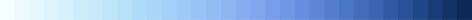Greens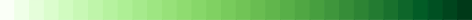Grays
Oranges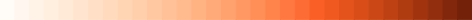Purples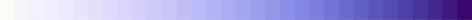Reds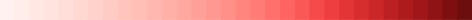#### Diverging

Name Example
RdBu (from red to blue)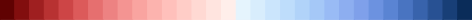It is also possible to create your own colormaps by using the `sequential_palette(h, [N::Int=100; c=0.88, s=0.6, b=0.75, w=0.15, d=0.0, wcolor=RGB(1,1,0), dcolor=RGB(0,0,1), logscale=false])`

function that creates a sequential map for a hue `h` (defined in LCHuv space). Other possible parameters that you can fine-tune are:

• `N` - number of colors
• `c` - the overall lightness contrast [0,1]
• `s` - saturation [0,1]
• `b` - brightness [0,1]
• `w` - cold/warm parameter, i.e. the strength of the starting color [0,1]
• `d` - depth of the ending color [0,1]
• `wcolor` - starting color (usually defined to be yellow)
• `dcolor` - ending color (depth)
• `logscale` - true/false for toggling logspacing

Two sequential maps can also be combined into a diverging colormap by using the

`diverging_palette(h1, h2 [, N::Int=100; mid=0.5,c=0.88, s=0.6, b=0.75, w=0.15, d1=0.0, d2=0.0, wcolor=RGB(1,1,0), dcolor1=RGB(1,0,0), dcolor2=RGB(0,0,1), logscale=false])`

where the arguments are

• `h1` - the main hue of the left side [0,360]
• `h2` - the main hue of the right side [0,360]

and optional arguments

• `N` - number of colors
• `c` - the overall lightness contrast [0,1]
• `s` - saturation [0,1]
• `b` - brightness [0,1]
• `w` - cold/warm parameter, i.e. the strength of the middle color [0,1]
• `d1` - depth of the ending color in the left side [0,1]
• `d2` - depth of the ending color in the right side [0,1]
• `wcolor` - starting color i.e. the middle color (warmness, usually defined to be yellow)
• `dcolor1` - ending color of the left side (depth)
• `dcolor2` - ending color of the right side (depth)
• `logscale` - true/false for toggling logspacing

# References

What perceptually uniform colorspaces are and why you should be using them:

Functions in this library were mostly implemented according to:

11/13/2012

11 months ago

189 commits